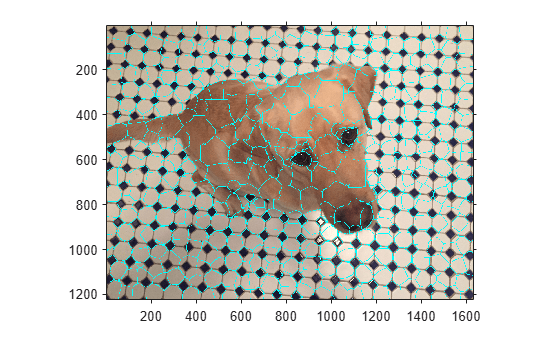# superpixels

2-D superpixel oversegmentation of images

## Syntax

``````[L,numLabels] = superpixels(A,N)``````
``````[L,numLabels] = superpixels(A,N,Name=Value)``````

## Description

example

``````[L,numLabels] = superpixels(A,N)``` computes superpixels of the 2-D grayscale or RGB image `A`. `N` specifies the number of superpixels you want to create. The function returns the label matrix `L` and the actual number of superpixels that were computed, `numLabels`.The `superpixels` function uses the simple linear iterative clustering (SLIC) algorithm . This algorithm groups pixels into regions with similar values. Using these regions in image processing operations, such as segmentation, can reduce the complexity of these operations.```
``````[L,numLabels] = superpixels(A,N,Name=Value)``` computes superpixels of image `A` using name-value arguments used to control aspects of the segmentation.```

## Examples

collapse all

`A = imread('kobi.png');`

Calculate superpixels of the image.

`[L,N] = superpixels(A,500);`

Display the superpixel boundaries overlaid on the original image.

```figure BW = boundarymask(L); imshow(imoverlay(A,BW,'cyan'),'InitialMagnification',67)```Set the color of each pixel in the output image to the mean RGB color of the superpixel region.

```outputImage = zeros(size(A),'like',A); idx = label2idx(L); numRows = size(A,1); numCols = size(A,2); for labelVal = 1:N redIdx = idx{labelVal}; greenIdx = idx{labelVal}+numRows*numCols; blueIdx = idx{labelVal}+2*numRows*numCols; outputImage(redIdx) = mean(A(redIdx)); outputImage(greenIdx) = mean(A(greenIdx)); outputImage(blueIdx) = mean(A(blueIdx)); end figure imshow(outputImage,'InitialMagnification',67)```## Input Arguments

collapse all

Image to segment, specified as a 2-D grayscale image or 2-D truecolor image. For `int16` data, `A` must be a grayscale image. When the parameter `isInputLab` is `true`, the input image must be data type `single` or `double`.

Data Types: `single` | `double` | `int16` | `uint8` | `uint16`

Desired number of superpixels, specified as a positive integer.

Data Types: `single` | `double` | `int8` | `int16` | `int32` | `int64` | `uint8` | `uint16` | `uint32` | `uint64`

### Name-Value Arguments

Specify optional pairs of arguments as `Name1=Value1,...,NameN=ValueN`, where `Name` is the argument name and `Value` is the corresponding value. Name-value arguments must appear after other arguments, but the order of the pairs does not matter.

Example: `B = superpixels(A,100,NumIterations=20);` performs twenty iterations.

Before R2021a, use commas to separate each name and value, and enclose `Name` in quotes.

Example: `B = superpixels(A,100,"NumIterations",20);`

Shape of superpixels, specified as a positive number. The compactness parameter of the SLIC algorithm controls the shape of superpixels. A higher value makes superpixels more regularly shaped, that is, a square. A lower value makes superpixels adhere to boundaries better, making them irregularly shaped. Typical values for compactness are in the range [1, 20].

Data Types: `single` | `double` | `int8` | `int16` | `int32` | `int64` | `uint8` | `uint16` | `uint32` | `uint64`

Input image data is in the L*a*b* color space, specified as `true` or `false`.

Data Types: `single` | `double` | `int8` | `int16` | `int32` | `int64` | `uint8` | `uint16` | `uint32` | `uint64` | `logical`

Algorithm used to compute superpixels, specified as one of the following values. The `superpixels` function uses two variations of the simple linear iterative clustering (SLIC) algorithm.

Value

Meaning

`"slic0"`

`superpixels` uses the SLIC0 algorithm to refine `Compactness` adaptively after the first iteration. This is the default.

`"slic"`

`Compactness` is constant during clustering.

Data Types: `char` | `string`

Number of iterations used in the clustering phase of the algorithm, specified as a positive integer. For most problems, it is not necessary to adjust this parameter.

Data Types: `single` | `double` | `int8` | `int16` | `int32` | `int64` | `uint8` | `uint16` | `uint32` | `uint64`

## Output Arguments

collapse all

Label matrix, returned as an array of positive integers. The value `1` indicates the first region, `2` the second region, and so on for each superpixel region in the image.

Data Types: `double`

Number of superpixels computed, returned as a positive integer.

Data Types: `double`

 Radhakrishna Achanta, Appu Shaji, Kevin Smith, Aurelien Lucchi, Pascal Fua, and Sabine Susstrunk, SLIC Superpixels Compared to State-of-the-art Superpixel Methods. IEEE Transactions on Pattern Analysis and Machine Intelligence, Volume 34, Issue 11, pp. 2274-2282, May 2012